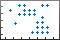# spy

Visualize sparsity pattern of matrix

•## Syntax

``spy(S)``
``spy(S,LineSpec)``
``spy(___,MarkerSize)``

## Description

example

````spy(S)` plots the sparsity pattern of matrix `S`. Nonzero values are colored while zero values are white. The plot displays the number of nonzeros in the matrix, `nz = nnz(S)`.```

example

````spy(S,LineSpec)` additionally specifies `LineSpec` to give the marker symbol and color to use in the plot. For example, `spy(A,'r*')` uses red asterisks for nonzeros.```

example

````spy(___,MarkerSize)` specifies `MarkerSize` to give the size of the markers using either of the previous input argument combinations.```

## Examples

collapse all

Plot the 60-by-60 sparse adjacency matrix of the connectivity graph of the Buckminster Fuller geodesic dome. This matrix also represents the carbon-60 molecule and a soccer ball.

```B = bucky; spy(B)```Specify the color and marker.

`spy(B,'ro')`Also specify the marker size.

`spy(B,'ro',2)`## Input Arguments

collapse all

Input matrix. `S` is typically a sparse matrix, but can be either full or sparse.

Data Types: `single` | `double` | `int8` | `int16` | `int32` | `int64` | `uint8` | `uint16` | `uint32` | `uint64` | `logical`
Complex Number Support: Yes

Size of markers, specified as a positive integer scalar.

Example: `spy(A,3)` uses markers of size 3.

Marker symbol and color, specified as a character vector or string containing symbols. The symbols can appear in any order. You do not need to specify both characteristics (marker and color). For example, if you omit the color and specify the marker, then the plot uses the default colors but uses the specified markers.

Example: `'or'` uses red circle markers.

MarkerDescriptionResulting Marker
`"o"`Circle`"+"`Plus sign`"*"`Asterisk`"."`Point`"x"`Cross`"_"`Horizontal line`"|"`Vertical line`"square"`Square`"diamond"`Diamond`"^"`Upward-pointing triangle`"v"`Downward-pointing triangle`">"`Right-pointing triangle`"<"`Left-pointing triangle`"pentagram"`Pentagram`"hexagram"`HexagramColor NameShort NameAppearance
`'red'``'r'``'green'``'g'``'blue'``'b'``'cyan'` `'c'``'magenta'``'m'``'yellow'``'y'``'black'``'k'``'white'``'w'`## Tips

• `format +` is a text-based alternative for displaying the nonzero structure of a small matrix:

```format + eye(4)```
```ans = + + + +```

## Version History

Introduced before R2006a

expand all